# K-Means Clustering¶

Let's check out the Stone Flakes data set. Find and download the StoneFlakes.dat file by clicking here or by running wget like below.

In :
!wget http://archive.ics.uci.edu/ml/machine-learning-databases/00299/StoneFlakes.dat

--2019-04-25 14:59:09--  http://archive.ics.uci.edu/ml/machine-learning-databases/00299/StoneFlakes.dat
Resolving archive.ics.uci.edu (archive.ics.uci.edu)... 128.195.10.252
Connecting to archive.ics.uci.edu (archive.ics.uci.edu)|128.195.10.252|:80... connected.
HTTP request sent, awaiting response... 200 OK
Length: 3523 (3.4K) [application/x-httpd-php]
Saving to: ‘StoneFlakes.dat’

StoneFlakes.dat     100%[===================>]   3.44K  --.-KB/s    in 0s

2019-04-25 14:59:09 (180 MB/s) - ‘StoneFlakes.dat’ saved [3523/3523]



Let's look at the first few lines.

In :
!head StoneFlakes.dat





Read about the column names and the meaning of the ID values at the data set's web site.

Notice that values are separated by commas, except for the first column. Also notice that there are question marks where data is missing. How can we read this? Well, the usual answer is to "google" for the answer. Try seaching for "read data set numpy"

Here we will use the pandas package to deal with these issues.

In :
import pandas

In :
d = pandas.read_csv(open('StoneFlakes.dat'))

In :
d[:5]

Out:
ID LBI RTI WDI FLA PSF FSF ZDF1 PROZD
ar ? 35.3 2.60 ? 42.4 24.2 47.1 69
arn 1.23 27.0 3.59 122 0.0 40.0 40.0 30
be 1.24 26.5 2.90 121 16.0 20.7 29.7 72
bi1 1.07 29.1 3.10 114 44.0 2.6 26.3 68
bi2 1.08 43.7 2.40 105 32.6 5.8 10.7 42
In :
d = pandas.read_csv(open('StoneFlakes.dat'), sep=',')
d[:5]

Out:
ID LBI RTI WDI FLA PSF FSF ZDF1 PROZD
ar ? 35.3 2.60 ? 42.4 24.2 47.1 69
arn 1.23 27.0 3.59 122 0.0 40.0 40.0 30
be 1.24 26.5 2.90 121 16.0 20.7 29.7 72
bi1 1.07 29.1 3.10 114 44.0 2.6 26.3 68
bi2 1.08 43.7 2.40 105 32.6 5.8 10.7 42

Let's just replace commas with spaces, using unix. Read about the tr unix command at Linux TR Command Examples

In :
! tr -s ' ' ',' < StoneFlakes.dat > StoneFlakes2.dat
! head StoneFlakes2.dat




In :
d = pandas.read_csv(open('StoneFlakes2.dat'))
d[:5]

Out:
ID LBI RTI WDI FLA PSF FSF ZDF1 PROZD
0 ar ? 35.3 2.60 ? 42.4 24.2 47.1 69
1 arn 1.23 27.0 3.59 122 0.0 40.0 40.0 30
2 be 1.24 26.5 2.90 121 16.0 20.7 29.7 72
3 bi1 1.07 29.1 3.10 114 44.0 2.6 26.3 68
4 bi2 1.08 43.7 2.40 105 32.6 5.8 10.7 42
In :
d = pandas.read_csv(open('StoneFlakes2.dat'), na_values='?')
d[:5]

Out:
ID LBI RTI WDI FLA PSF FSF ZDF1 PROZD
0 ar NaN 35.3 2.60 NaN 42.4 24.2 47.1 69
1 arn 1.23 27.0 3.59 122.0 0.0 40.0 40.0 30
2 be 1.24 26.5 2.90 121.0 16.0 20.7 29.7 72
3 bi1 1.07 29.1 3.10 114.0 44.0 2.6 26.3 68
4 bi2 1.08 43.7 2.40 105.0 32.6 5.8 10.7 42
In :
d = pandas.read_csv(open('StoneFlakes2.dat'), na_values='?', error_bad_lines=False)
d[:5]

Out:
ID LBI RTI WDI FLA PSF FSF ZDF1 PROZD
0 ar NaN 35.3 2.60 NaN 42.4 24.2 47.1 69
1 arn 1.23 27.0 3.59 122.0 0.0 40.0 40.0 30
2 be 1.24 26.5 2.90 121.0 16.0 20.7 29.7 72
3 bi1 1.07 29.1 3.10 114.0 44.0 2.6 26.3 68
4 bi2 1.08 43.7 2.40 105.0 32.6 5.8 10.7 42
In :
d[:5].isnull()

Out:
ID LBI RTI WDI FLA PSF FSF ZDF1 PROZD
0 False True False False True False False False False
1 False False False False False False False False False
2 False False False False False False False False False
3 False False False False False False False False False
4 False False False False False False False False False
In :
d[:5].isnull().any(axis=1)

Out:
0     True
1    False
2    False
3    False
4    False
dtype: bool
In :
d[:5].isnull().any(axis=1) == False

Out:
0    False
1     True
2     True
3     True
4     True
dtype: bool
In :
print(d.shape)
d = d[d.isnull().any(axis=1)==False]
print(d.shape)

(79, 9)
(73, 9)

In :
import numpy as np
import matplotlib.pyplot as plt
%matplotlib inline

In :
data = d.iloc[:,1:].values
data.shape

Out:
(73, 8)
In :
data[:5,:]

Out:
array([[  1.23,  27.  ,   3.59, 122.  ,   0.  ,  40.  ,  40.  ,  30.  ],
[  1.24,  26.5 ,   2.9 , 121.  ,  16.  ,  20.7 ,  29.7 ,  72.  ],
[  1.07,  29.1 ,   3.1 , 114.  ,  44.  ,   2.6 ,  26.3 ,  68.  ],
[  1.08,  43.7 ,   2.4 , 105.  ,  32.6 ,   5.8 ,  10.7 ,  42.  ],
[  1.39,  29.5 ,   2.78, 126.  ,  14.  ,   0.  ,  50.  ,  78.  ]])

To see this data, let's try plotting each column as a separate curve on the same axes.

In :
plt.plot(data);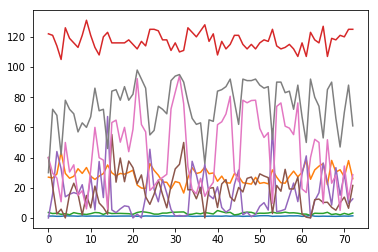Each sample has 8 attributes, so each sample is a point in 8-dimensional space. I wonder how well the samples "clump" in those 8 dimensions. Let's try clustering them with the k-means algorithm.

First, let's try to find two clusters, so $k=2$. We must initialize the two means of the two clusters. Let's just pick two samples at random.

In :
np.random.choice(range(data.shape),2, replace=False) # data.shape is number of rows, or samples

Out:
array([18, 36])
In :
np.random.choice(range(data.shape),2, replace=False)

Out:
array([70, 49])
In :
centersIndex = np.random.choice(range(data.shape),2, replace=False)
centersIndex

Out:
array([31,  2])
In :
centers = data[centersIndex,:]
centers

Out:
array([[  1.21,  23.3 ,   3.9 , 110.  ,   1.6 ,  35.5 ,  94.1 ,  95.  ],
[  1.07,  29.1 ,   3.1 , 114.  ,  44.  ,   2.6 ,  26.3 ,  68.  ]])

Now we must find all samples that are closest to the first center, and those that are closest to the second sample.

In :
a = np.array([1,2,3])
b = np.array([10,20,30])
a, b

Out:
(array([1, 2, 3]), array([10, 20, 30]))
In :
a-b

Out:
array([ -9, -18, -27])

But what if we want to subtract every element of a with every element of b?

In :
np.resize(a,(3,3))

Out:
array([[1, 2, 3],
[1, 2, 3],
[1, 2, 3]])
In :
np.resize(b, (3,3))

Out:
array([[10, 20, 30],
[10, 20, 30],
[10, 20, 30]])
In :
np.resize(a,(3,3)).T

Out:
array([[1, 1, 1],
[2, 2, 2],
[3, 3, 3]])
In :
np.resize(a,(3,3)).T - np.resize(b,(3,3))

Out:
array([[ -9, -19, -29],
[ -8, -18, -28],
[ -7, -17, -27]])

However, we can ask numpy to do this duplication for us if we reshape a to be a column vector and leave b as a row vector.

$$\begin{pmatrix} 1\\ 2\\ 3 \end{pmatrix} - \begin{pmatrix} 10 & 20 & 30 \end{pmatrix} \;\; = \;\; \begin{pmatrix} 1 & 1 & 1\\ 2 & 2 & 2\\ 3 & 3 & 3 \end{pmatrix} - \begin{pmatrix} 10 & 20 & 30\\ 10 & 20 & 30\\ 10 & 20 & 30 \end{pmatrix}$$
In :
a = a.reshape((-1,1))
a

Out:
array([,
,
])
In :
a - b

Out:
array([[ -9, -19, -29],
[ -8, -18, -28],
[ -7, -17, -27]])
In :
a = np.array([1,2,3])
b = np.array([[10,20,30],[40,50,60]])
print(a)
print(b)

[1 2 3]
[[10 20 30]
[40 50 60]]

In :
b-a

Out:
array([[ 9, 18, 27],
[39, 48, 57]])

The single row vector a is duplicated for as many rows as there are in b! We can use this to calculate the squared distance between a center and every sample.

In :
centers[0,:]

Out:
array([  1.21,  23.3 ,   3.9 , 110.  ,   1.6 ,  35.5 ,  94.1 ,  95.  ])
In :
np.sum((centers[0,:] - data)**2, axis=1)

Out:
array([ 7332.4065,  5235.0009,  8256.3096, 12051.0769,  3943.5468,
5270.79  ,  4587.1536,  8387.04  ,  8514.841 ,  9991.3525,
6339.5253,  1478.7909,  5474.2501,  4741.9944, 15881.0021,
1562.1049,  1159.3125,  2328.4601,  1469.5104,  2961.5116,
1525.7004,   183.24  ,  1158.7321,  2103.8749, 10376.8525,
7679.526 ,  5631.84  ,  6094.28  ,  6037.1525,   723.8609,
163.45  ,     0.    ,   624.9065,  4490.7444,  8351.9097,
8590.9589,  6329.6436, 12702.2969,  6968.2309,  6479.5601,
2349.2509,  1289.6581,   810.1481,   700.5381,  5537.8345,
7283.9726,   628.5424,   428.3436,   378.7449,   469.2709,
1373.7622,  1941.9369,  1618.1716, 13650.1561,   665.4716,
676.2249,  1289.6901,  1790.7189,  2833.7069,   551.1824,
8330.0989, 11030.5916,  4378.158 ,  2769.4949,  3300.6525,
11240.3225,  3018.4445,  6242.8469,  7228.7389, 11270.9338,
5532.2509,  7979.2461,  5996.028 ])
In :
np.sum((centers[1,:] - data)**2, axis=1) > np.sum((centers[0,:] - data)**2, axis=1)

Out:
array([False, False, False, False, False, False, False, False, False,
False, False,  True, False, False, False,  True,  True,  True,
True,  True,  True,  True,  True,  True, False, False, False,
False, False,  True,  True,  True,  True, False, False, False,
False, False, False, False, False,  True,  True,  True, False,
False,  True,  True,  True,  True,  True,  True,  True, False,
True,  True,  True,  True, False,  True, False, False, False,
False, False, False, False, False, False, False, False, False,
False])
In :
centers

Out:
array([[  1.21,  23.3 ,   3.9 , 110.  ,   1.6 ,  35.5 ,  94.1 ,  95.  ],
[  1.07,  29.1 ,   3.1 , 114.  ,  44.  ,   2.6 ,  26.3 ,  68.  ]])
In :
centers[:,np.newaxis,:].shape, data.shape

Out:
((2, 1, 8), (73, 8))
In :
(centers[:,np.newaxis,:] - data).shape

Out:
(2, 73, 8)
In :
np.sum((centers[:,np.newaxis,:] - data)**2, axis=2).shape

Out:
(2, 73)
In :
np.argmin(np.sum((centers[:,np.newaxis,:] - data)**2, axis=2), axis=0)

Out:
array([1, 1, 1, 1, 1, 1, 1, 1, 1, 1, 1, 0, 1, 1, 1, 0, 0, 0, 0, 0, 0, 0,
0, 0, 1, 1, 1, 1, 1, 0, 0, 0, 0, 1, 1, 1, 1, 1, 1, 1, 1, 0, 0, 0,
1, 1, 0, 0, 0, 0, 0, 0, 0, 1, 0, 0, 0, 0, 1, 0, 1, 1, 1, 1, 1, 1,
1, 1, 1, 1, 1, 1, 1])
In :
cluster = np.argmin(np.sum((centers[:,np.newaxis,:] - data)**2, axis=2), axis=0)
cluster

Out:
array([1, 1, 1, 1, 1, 1, 1, 1, 1, 1, 1, 0, 1, 1, 1, 0, 0, 0, 0, 0, 0, 0,
0, 0, 1, 1, 1, 1, 1, 0, 0, 0, 0, 1, 1, 1, 1, 1, 1, 1, 1, 0, 0, 0,
1, 1, 0, 0, 0, 0, 0, 0, 0, 1, 0, 0, 0, 0, 1, 0, 1, 1, 1, 1, 1, 1,
1, 1, 1, 1, 1, 1, 1])
In :
data[cluster==0,:].mean(axis=0)

Out:
array([  1.22241379,  24.63758621,   3.40344828, 114.17241379,
5.17241379,  27.41724138,  68.14482759,  88.        ])
In :
data[cluster==1,:].mean(axis=0)

Out:
array([  1.19818182,  30.32954545,   2.87340909, 119.52272727,
23.14772727,  11.83636364,  27.325     ,  65.86363636])
In :
k = 2
for i in range(k):
centers[i,:] = data[cluster==i,:].mean(axis=0)

In :
centers

Out:
array([[  1.22241379,  24.63758621,   3.40344828, 114.17241379,
5.17241379,  27.41724138,  68.14482759,  88.        ],
[  1.19818182,  30.32954545,   2.87340909, 119.52272727,
23.14772727,  11.83636364,  27.325     ,  65.86363636]])
In :
def kmeans(data, k = 2, n = 5):
# Initial centers
centers = data[np.random.choice(range(data.shape), k, replace=False), :]
# Repeat n times
for iteration in range(n):
# Which center is each sample closest to?
closest = np.argmin(np.sum((centers[:, np.newaxis, :] - data)**2, axis=2), axis=0)
# Update cluster centers
for i in range(k):
centers[i, :] = data[closest==i, :].mean(axis=0)
return centers

In :
kmeans(data,2)

Out:
array([[  1.23194444,  25.36083333,   3.36833333, 114.47222222,
7.20833333,  23.78333333,  64.91944444,  86.58333333],
[  1.18432432,  30.7027027 ,   2.8072973 , 120.24324324,
24.56756757,  12.42432432,  22.74054054,  63.05405405]])
In :
kmeans(data,2)

Out:
array([[  1.18432432,  30.7027027 ,   2.8072973 , 120.24324324,
24.56756757,  12.42432432,  22.74054054,  63.05405405],
[  1.23194444,  25.36083333,   3.36833333, 114.47222222,
7.20833333,  23.78333333,  64.91944444,  86.58333333]])

Let's define $J$ as the performance measure being minimized by k-means. $$J = \sum_{n=1}^N \sum_{k=1}^K r_{nk} ||\mathbf{x}_n - \mathbf{\mu}_k||^2$$ where $N$ is the number of samples, $K$ is the number of cluster centers, $\mathbf{x}_n$ is the $n^{th}$ sample and $\mathbf{\mu}_k$ is the $k^{th}$ center, each being an element of $\mathbf{R}^p$ where $p$ is the dimensionality of the data.

The sums can be computed using python for loops, but for loops are much slower than matrix operations in python, as the following cells show.

In :
a = np.linspace(0,10,30).reshape(3,10)
a

Out:
array([[ 0.        ,  0.34482759,  0.68965517,  1.03448276,  1.37931034,
1.72413793,  2.06896552,  2.4137931 ,  2.75862069,  3.10344828],
[ 3.44827586,  3.79310345,  4.13793103,  4.48275862,  4.82758621,
5.17241379,  5.51724138,  5.86206897,  6.20689655,  6.55172414],
[ 6.89655172,  7.24137931,  7.5862069 ,  7.93103448,  8.27586207,
8.62068966,  8.96551724,  9.31034483,  9.65517241, 10.        ]])
In :
b = np.arange(30).reshape(3,10)
b

Out:
array([[ 0,  1,  2,  3,  4,  5,  6,  7,  8,  9],
[10, 11, 12, 13, 14, 15, 16, 17, 18, 19],
[20, 21, 22, 23, 24, 25, 26, 27, 28, 29]])
In :
result = np.zeros((3,10))
for i in range(3):
for j in range(10):
result[i,j] = a[i,j] + b[i,j]
result

Out:
array([[ 0.        ,  1.34482759,  2.68965517,  4.03448276,  5.37931034,
6.72413793,  8.06896552,  9.4137931 , 10.75862069, 12.10344828],
[13.44827586, 14.79310345, 16.13793103, 17.48275862, 18.82758621,
20.17241379, 21.51724138, 22.86206897, 24.20689655, 25.55172414],
[26.89655172, 28.24137931, 29.5862069 , 30.93103448, 32.27586207,
33.62068966, 34.96551724, 36.31034483, 37.65517241, 39.        ]])
In :
a.shape, a[:,np.newaxis].shape

Out:
((3, 10), (3, 1, 10))
In :
%%timeit
result = np.zeros((3,10))
for i in range(3):
for j in range(10):
result[i,j] = a[i,j] + b[i,j]

17.8 µs ± 234 ns per loop (mean ± std. dev. of 7 runs, 100000 loops each)

In :
result = a + b
result

Out:
array([[ 0.        ,  1.34482759,  2.68965517,  4.03448276,  5.37931034,
6.72413793,  8.06896552,  9.4137931 , 10.75862069, 12.10344828],
[13.44827586, 14.79310345, 16.13793103, 17.48275862, 18.82758621,
20.17241379, 21.51724138, 22.86206897, 24.20689655, 25.55172414],
[26.89655172, 28.24137931, 29.5862069 , 30.93103448, 32.27586207,
33.62068966, 34.96551724, 36.31034483, 37.65517241, 39.        ]])
In :
%%timeit
result = a + b

1.09 µs ± 16.5 ns per loop (mean ± std. dev. of 7 runs, 1000000 loops each)


So, the matrix form is 10 times faster!

Now, back to our problem. How can we use matrix operations to calculate the squared distance between two centers and, say, five data samples? Let's say both are two-dimensional.

In :
centers = np.array([[1,2],[5,4]])
centers

Out:
array([[1, 2],
[5, 4]])
In :
data = np.array([[3,2],[4,6],[7,3],[4,6],[1,8]])
data

Out:
array([[3, 2],
[4, 6],
[7, 3],
[4, 6],
[1, 8]])

This will be a little weird, and hard to understand, but by adding an empty dimension to the centers array, numpy broadcasting does all the work for us.

In :
centers[:,np.newaxis,:]

Out:
array([[[1, 2]],

[[5, 4]]])
In :
centers[:,np.newaxis,:].shape

Out:
(2, 1, 2)
In :
data.shape

Out:
(5, 2)
In :
diffsq = (centers[:,np.newaxis,:] - data)**2
diffsq

Out:
array([[[ 4,  0],
[ 9, 16],
[36,  1],
[ 9, 16],
[ 0, 36]],

[[ 4,  4],
[ 1,  4],
[ 4,  1],
[ 1,  4],
[16, 16]]])
In :
diffsq.shape

Out:
(2, 5, 2)
In :
np.sum(diffsq,axis=2)

Out:
array([[ 4, 25, 37, 25, 36],
[ 8,  5,  5,  5, 32]])

Now we have a 2 x 5 array with the first row containing the squared distance from the first center to each of the five data samples, and the second row containing the squared distances from the second center to each of the five data samples.

Now we just have to find the smallest distance in each column and sum them up.

In :
np.min(np.sum(diffsq,axis=2), axis=0)

Out:
array([ 4,  5,  5,  5, 32])
In :
np.sum(np.min(np.sum(diffsq,axis=2), axis=0))

Out:
51

Let's define a function named calcJ to do this calculation.

In :
def calcJ(data,centers):
diffsq = (centers[:,np.newaxis,:] - data)**2
return np.sum(np.min(np.sum(diffsq,axis=2), axis=0))

In :
calcJ(data,centers)

Out:
51
In :
def kmeans(data, k = 2, n = 5):
# Initialize centers and list J to track performance metric
centers = data[np.random.choice(range(data.shape),k,replace=False), :]
J = []

# Repeat n times
for iteration in range(n):

# Which center is each sample closest to?
sqdistances = np.sum((centers[:,np.newaxis,:] - data)**2, axis=2)
closest = np.argmin(sqdistances, axis=0)

# Calculate J and append to list J
J.append(calcJ(data,centers))

# Update cluster centers
for i in range(k):
centers[i,:] = data[closest==i,:].mean(axis=0)

# Calculate J one final time and return results
J.append(calcJ(data,centers))
return centers, J, closest

In :
centers,J,closest = kmeans(data,2)

In :
J

Out:
[30, 19, 19, 19, 19, 19]
In :
plt.plot(J);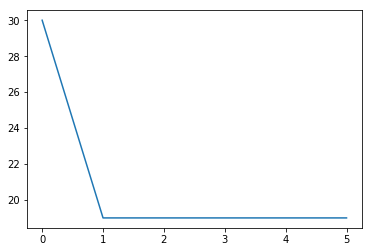In :
centers,J,closest = kmeans(data, 2, 10)
plt.plot(J);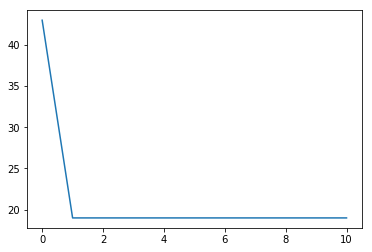In :
centers,J,closest = kmeans(data, 3, 10)
plt.plot(J);

/s/parsons/e/fac/anderson/anaconda3/lib/python3.6/site-packages/ipykernel_launcher.py:18: RuntimeWarning: Mean of empty slice.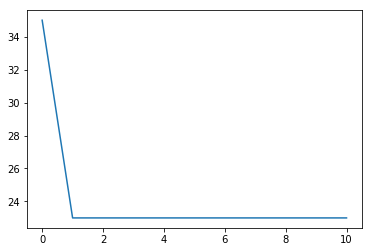In :
small = np.array([[8,7],[7,6.6],[9.2,8.3],[6.8,9.2], [1.2,3.2],[4.8,2.3],[3.4,3.2],[3.2,5.6],[1,4],[2,2.2]])

In :
plt.scatter(small[:,0],small[:,1]);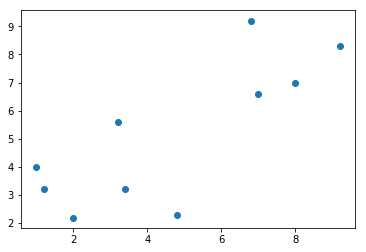In :
c,J,closest = kmeans(small,2,n=2)

In :
c

Out:
array([[2.48, 2.98],
[6.84, 7.34]])
In :
closest

Out:
array([1, 1, 1, 1, 0, 0, 0, 1, 0, 0])
In :
plt.scatter(small[:,0], small[:,1], s=80, c=closest, alpha=0.5);
plt.scatter(c[:,0],c[:,1],s=80,c="green",alpha=0.5);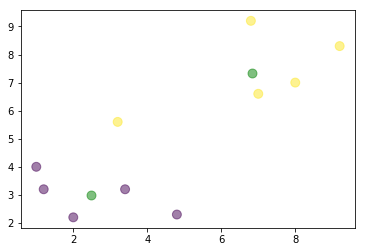In :
c,J,closest = kmeans(small,2,n=2)
plt.scatter(small[:,0], small[:,1], s=80, c=closest, alpha=0.5);
plt.scatter(c[:,0],c[:,1],s=80,c="green",alpha=0.5);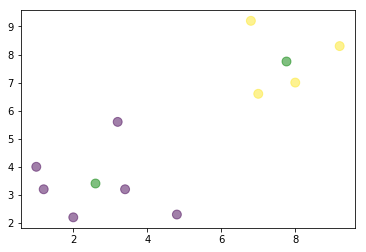In :
J

Out:
[48.12, 26.565833333333334, 26.565833333333334]
In :
import gzip
import pickle

with gzip.open('mnist.pkl.gz', 'rb') as f:
train_set, valid_set, test_set = pickle.load(f, encoding='latin1')
# zero = train_set[1,:].reshape((28,28,1))
# one = train_set[3,:].reshape((28,28,1))
# two = train_set[5,:].reshape((28,28,1))
# four = train_set[20,:].reshape((28,28,1))

X = train_set
T = train_set.reshape((-1,1))

Xtest = test_set
Ttest = test_set.reshape((-1,1))

X.shape, T.shape, Xtest.shape, Ttest.shape

Out:
((50000, 784), (50000, 1), (10000, 784), (10000, 1))
In :
c,J,closest = kmeans(X, k=10, n=20)

In :
plt.plot(J)

Out:
[<matplotlib.lines.Line2D at 0x7fb74869d710>]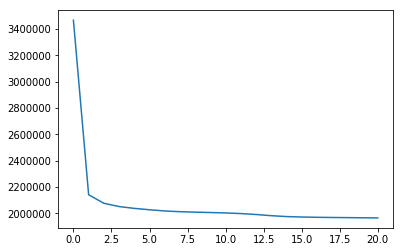In :
c.shape

Out:
(10, 784)
In :
for i in range(10):
plt.subplot(2,5,i+1)
plt.imshow(-c[i,:].reshape((28,28)), interpolation='nearest', cmap='gray')
plt.axis('off')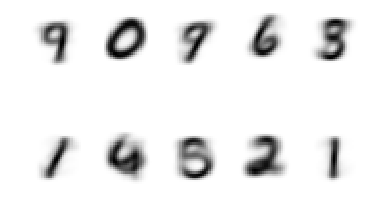In :
c, J, closest = kmeans(X, k=10, n=20)
plt.plot(J)
plt.figure()
for i in range(10):
plt.subplot(2, 5, i+1)
plt.imshow(-c[i, :].reshape((28, 28)), interpolation='nearest', cmap='gray')
plt.axis('off')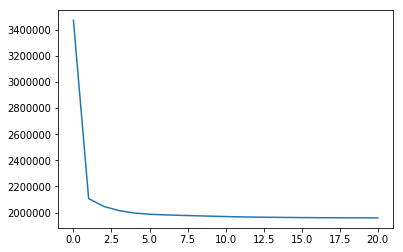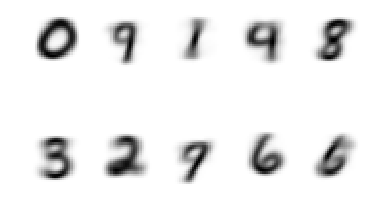In :
c,J,closest = kmeans(X, k=20, n=20)
plt.plot(J)
plt.figure()
for i in range(20):
plt.subplot(4,5,i+1)
plt.imshow(-c[i,:].reshape((28,28)), interpolation='nearest', cmap='gray')
plt.axis('off')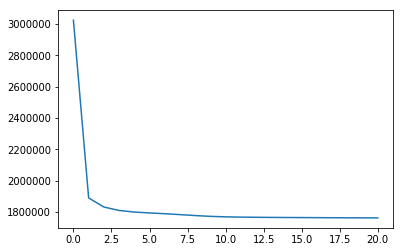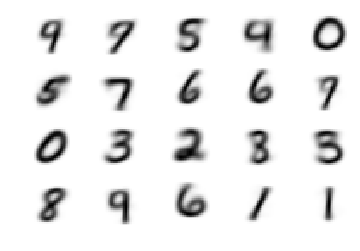In :
c,J,closest = kmeans(X, k=20, n=30)
plt.plot(J)
plt.figure()
for i in range(20):
plt.subplot(4,5,i+1)
plt.imshow(-c[i,:].reshape((28,28)), interpolation='nearest', cmap='gray')
plt.axis('off')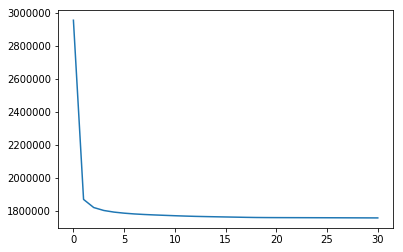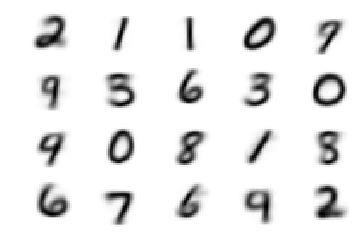In [ ]: